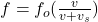Question

________ In many cartoon shows, a character runs off a cliff, realizes his predicament, and lets out a scream. He continues to scream as he falls. If the physical situation is portrayed correctly, from the vantage point of an observer at the top of the cliff leaning over the edge, the pitch of the scream as he falls should be A) higher than the original pitch and constant. B) higher than the original pitch and increasing as he falls. C) lower than the original pitch and constant. D) lower than the original pitch and decreasing as he falls. E) It is impossible to predict

1.Here the source is moving away from the observer so frequency will be smaller than the actual frequency and since the speed is increasing so the frequency is decreasing with time so correct answer is

D) lower than the original pitch and decreasing as he falls.

Explanation:

As we know by the Doppler’s effect of sound we have

so we will haveso here when source moves away from the observer with a some speed then the frequency of the sound observed by the observer is smaller than the actual frequency

Here we know that the speed of the source is increasing with time as the source is falling under gravity

So we can say that the pitch of the sound will decrease with time